# 3 ways to scale an analog input signal

Other Parts Discussed in Post: TLV341A, TMUX1308, ADS7040

As electronic devices become more self-aware, the need for voltage scaling increases. I’m not talking about artificial intelligence, like Hal from “2001: A Space Odyssey. I’m referring to are electronic devices that have more self-checks, which entail reading many voltages of various ranges.

Scaling an input voltage isn’t always as easy (or complex) as it first seems. In this post, I’ll walk through how I tackled this challenge in a recent signal chain design that needed to scale a +/-10-V signal down to a 0 to 2.5 V range to match all the other signals headed to the analog-to-digital converter (ADC).  The transfer function to do this is linear: VOUT = VIN/8 + 1.25V.

Note: If you are looking for a solution for a specific voltage scaling need, visit this TI E2ETM support FAQ. It includes an Excel spreadsheet calculator that provides a circuit with ideal component values for just about any inverting or noninverting voltage scaling task.

Solution #1:

My first thought was to use a noninverting operational amplifier (op amp) circuit. After doing some quick math, I determined that the circuit, shown in Figure 1, needed a 1.43V offset supply and a feedback/ground-resistor ratio of -7/8.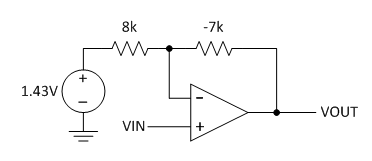Figure 1: Solution #1 simulates well, but is impossible to implement

The noninverting amplifier gain formula is (1 + RF/RG). For a gain of +1/8, the resistor ratio is negative. I can’t buy a -7k resistor, so that is big problem. My op amp will need an input common-mode range down to -10V; this is also a problem, because I don’t have a negative supply available. Clearly a noninverting op amp circuit is incompatible in this case, however it does work when the voltage gain needed is greater than one.

Solution #2:

The five-resistor op-amp circuit shown in Figure 2 is a difference amplifier with the inverting input grounded and the noninverting input terminated 1.25V. The gain is set to 1/8. The input common-mode range is 0V to 2.22V, so I can use a single-supply op amp.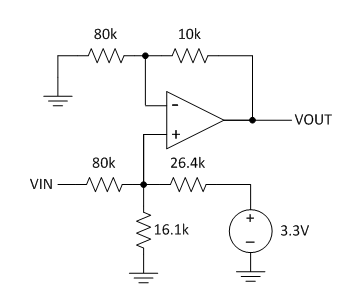Figure 2: Solution #2 works, but could there be a better solution?

Solution #3:

I don’t need an op amp to attenuate a signal. I can use three resistors – A, B and C – and a voltage source, V, to perform the desired scaling task. See Figure 3.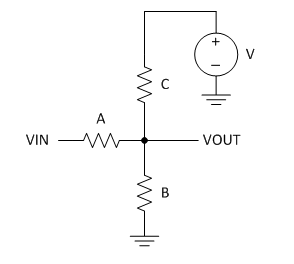Figure 3: This simple solution uses just three resistors with an existing power source

In my example, the gain is 1/8 and the offset is 1.25V. I’ll use the letters G and Z to represent gain and offset (output with zero input); thus, G = 1/8 and Z = 1.25V. My supply voltage, V, is 3.3V.

So what is the best way to solve for the values (or ratios) of resistors A, B and C? I could use the resistor-divider rule, VOUT = VIN * RI / (RG + RI), to calculate G and Z with Equations 1 and 2:

G = Gain = dVOUT/dVIN = (B||C) / (A+B||C)                    (1)

Z = Zero = VOUT[VIN=0V] = A||B / (C + A||B) * V                      (2)

The || symbol means “in parallel”; for example, x||y is x*y/(x+y) or 1/(1/x+1/y).

Solving these equations using the resistor-divider rule will be an ugly process, and it’s easy to make mistakes. I know – because I’ve done it.

A cleaner method involves using determinates to solve for three unknowns using three equations in the form of [ x1a +x2b + x3c = constant ].

To make my life easier, I changed resistance [A, B, C] into conductance [1/A, 1/B, 1/C] = [a, b, c].

I used Kirchhoff’s current law to create the first equation based on desired voltage gain. I set VIN=1VAC to make G = VOUT. See Figure 4. Equation 3 is the formula for AC current:

(G-1)*a + G*b + G*c = 0                               (3)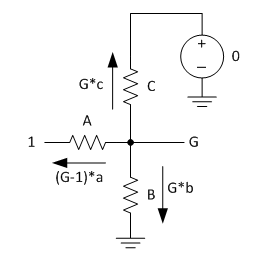Figure 4: Kirchhoff’s current schematic for equation 3

I used Kirchhoff’s current law to create the second equation based on desired voltage offset. I set VIN = 0V, VOUT = Z, which is the output voltage with 0V input, see Figure 5. Thus, Equation 4 is:

Z*a + Z*b + (Z-V)*c = 0                  (4)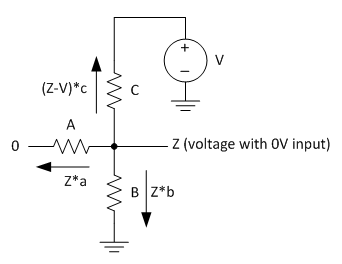Figure 5: Kirchhoff’s current schematic for equation 4

You’ll need a third equation before you can solve three equations in three unknowns. Just about any equation will do; for example, setting resistor A to 10k gives you Equation 5:

1*a + 0*b + 0*c = 1/10,000                           (5)

Now you can solve for all three resistors using determinates and convert the solved conductance [a, b, c] back to resistance [A, B, C] at the same time. Remember that G is gain and Z is output for 0V input, and V is the power-supply voltage. Figure 6 shows the solution using the three equations.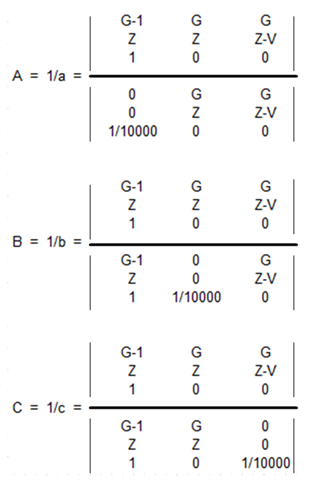Figure 6: Solution for the three equations

Solving determinates by hand can also lead to math errors, so let Microsoft Excel or some other math program do it for you. My solution was resistor [A, B, C] = [10k, 2.52k, 3.3k]. Rounded to the nearest 1%, the resistor is [10k, 2.55k, 3.3k].

If any resistance values come out negative, which indicates the the solution is not buildable, try changing the C resistor’s voltage supply (magnitude and polarity) and verify that the gain you need is less than 1.

Implementing solution 3 into a multiplexed channel ADC application:

Figure 7 is my final circuit that scales the +/10V signal going to channel 1. The schematic also includes a TMUX1308 8-channel input multiplexer, TLV341A amplifier/buffer, and a ADS7040 ADC.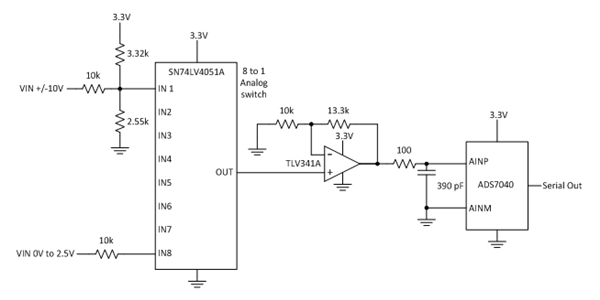Figure 7: Eight-channel analog scaling solution (two inputs shown)

The three-resistor solution is simple and accurate. However, keep in mind that the input impedance of the source signal and the load impedance placed on the output will become part of the scaler, and affect accuracy.

What’s your experience designing with resistors? Sign in and comment below.

Additional resources

• Antenna_Head, your comments are well founded. The main point of the blog is that five resistor solutions can often be solved with just three.

Best is when the divider voltage source is tied to the ADC reference so much of error will cancel (the ADS7040 uses the same 3.3V supply as its reference). For voltage source noise reduction, it is tempting to add a capacitor across the ground resistor 'B'. This works, but it also creates a low pass filter for the input signal. Adding a properly scaled capacitor across the input resistor ‘A’ can make the input signal frequency neutral again; at the cost of the input now being capacitive.

The solution calculates the ratio between the three resistors, so resistances can be increased in the same ratio as high as desired. Higher resistance will lower the low pass cutoff frequency caused by the parasitic capacitance (switch and op amp input capacitance).

• Interesting; a couple points of feedback.

1) this seems to assume a perfect 3.3V supply.  Voltage dividers have very poor PSRR, so any noise on the power supply, temperature drift, etc... will end up as (ratioed) noise and offset in the signal chain.  This is tough to overcome without a separate quiet/stable reference to provide the output common mode. Maybe good to add a hook into the reference for the ADC to help overcome this?

2) The input impedance of this network, as seen by the signal source, is quite low, on the order of 11.4k.  This loading might have undesirable affects depending on where the signal is coming from... just something to lookout for.

Thanks!

• This is a great article

• great article.

How you arrive to the (G-1)*a + G*b + G*c = 0

Regards, Alex

• Kailyn,

I started with the gain which is 1/8 so inverting feedback resistor is 1/8 of the inverting ground resistor. 10k and 80k were selected to match the desired ratio.

For the non-inverting resistors, I could have used the same resistors 10k and 80k if the reference voltage was 1.25V. Because I preferred to use 3.3V, I had to make a 2 resistor divider that created a Thevenin's equivalent voltage of 1.25V and resistance of 10k.

1.25V =  3.3V * (Rg / ( Rg + Rref) ) ;  1.2501V = 3.3V *16.1k / (16.1k +26.4k)

10k = Rg * Rref / (Rg +Rref);   10.001k = 16.1k *26.4k / (16.1k +26.4k)

To get those resistor values I rearranged the formulas

1.25V /3.3V  =  Rg / ( Rg + Rref)

10k / Rref  = Rg  / (Rg +Rref)

Therefore  1.25V / 3.3V = 10k / Rref

Rref = 10k *3.3V / 1.25V = 26.4k

Then I put known Rref into one the original equations and solved for Rg